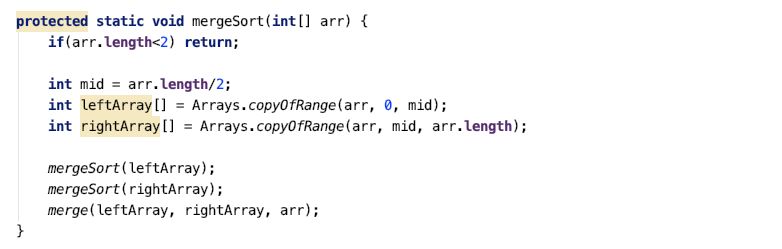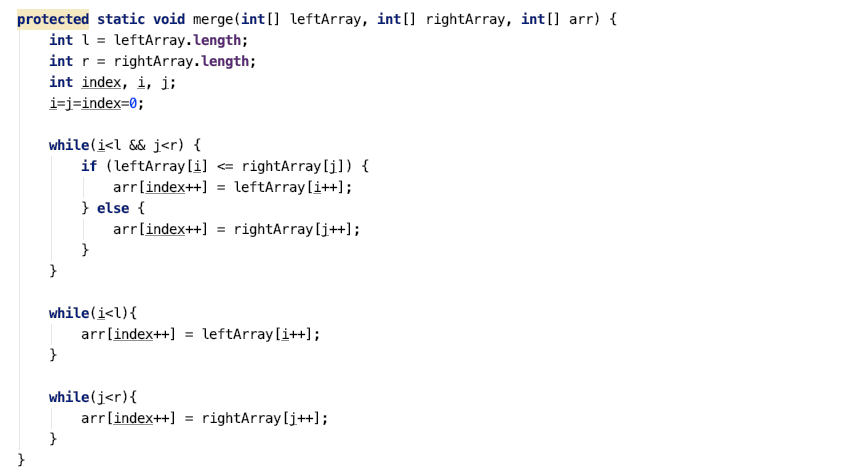# Merge Sort

Sorting is a technique used to arrange the collection of objects into either ascending order or descending order. There are many sorting algorithms, and merge sort is one of them.

According to Wikipedia, Merge sort is an efficient, general-purpose, comparison-based sorting algorithm. Most implementations produce a stable sort, which means that the order of equal elements is the same in the input and output. Merge sort is a divide and conquer algorithm that was invented by John von Neumann in 1945.

## Algorithm

Suppose, we are given with the above array and we want to sort it in the non-decreasing order. Let’s see how merge sort does it for us!

In each step of merge sort,

1. We recursively divide the array in two equal parts till it can’t be divided any further, i.e. till the array size is greater than equal to 1. Auxiliary arrays are created of smaller sizes.
2. After the divide step, we merge the smaller arrays such the resultant array is in the ascending order.

## Code AnalysisIn mergeSort function, if array size is greater than 1 then we are dividing the array in two parts by mid index. Then we are calling mergeSort for left part and the right part recursively. After dividing the array in left and right parts, we call the merge function.Merge function is merging left and right array in original array, arr. We start iterating over left and right array from left, i=0, j=0 and index=0. We select the smaller of the left[i] and right[j] and put it in the arr[index]. When left[i] is smaller, then copy left[i] to arr[index] and set i=i+1 and index=index+1. When right[j] is smaller, then copy right[j] to arr[index] and set j=j+1 and index=index+1

## Time Complexity

mergeSort = O(nlogn)

merge = O(n)

`Let's see how:T(n) = 2T(n/2) + c*nHere, T(n) is for merge sort for array of size n and 2T(n/2) for two merge sort calls with array of sizes n/2.T(n/2) = 2T(n/4)+ c*n/2 T(n/4) = 2T(n/8)+ c*n/4T(n) = 2(2T(n/4) + c*n/2)+c*n      = 4T(n/4)+2c*n      = 4(2T(n/8)+c*n/4)+2c*n      = 8T(n/8)+3c*nGeneralizing, T(n) = (2^k)T(n/(2^k)) + c*k*nFor, T(1) => n/(2^k) = 1 => n=2^k => k=lognT(n) = nT(1) + nlogn => O(nlogn)`

## Space Complexity

O(nlogn) for all the auxiliary arrays if there is no garbage collection and O(n) for the auxiliary array with garbage collection

Guys I really hope that you find this article valuable! If still you have any queries, you can surely discuss them in the comment section below.

Thanks a lot for devoting your time to this blog.

--

--